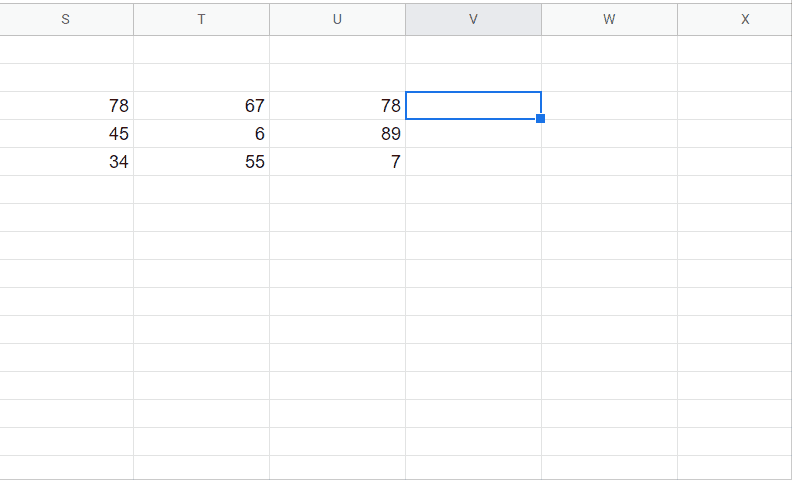# How to calculate standard deviation in Google Sheet

There is a built-in formula for calculating standard deviation of a dataset in Google sheets. The formula to achieve this goal is given below.

=STDEV(value1, [value2, …])

In this formula, select the first value and select the value for which you wish to calculate standard deviation. You can select a data range also. Steps are given below to calculate the standard deviation.Google sheet is a powerful tool for data calculation and data manipulations. Google sheets are enriched with a lot of functions and tools to conduct mathematical and statistical calculations.

### Step 1 – Select the cell and apply formulaAbove is an animation as an example.

– Select any cell and which you like to calculate standard deviation.
– Type the formula.
– Select the first cell and drag it to the last cell of your data range.
– Or simply type the first cell number followed by the colon(:) and last cell number of data range.
– Close the bracket.
– As soon as you press the enter key it will calculate standard deviation.